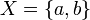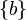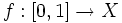# Sierpiński space

Jump to: navigation, search
This article is about a particular topological space (uniquely determined up to homeomorphism)|View a complete list of particular topological spaces

## Definition

### Explicit definition

The Sierpiński space is a topological space defined as follows (up to homeomorphism):

• The underlying set is a two-point set$X = \{ a,b \}$.
• The open subsets are:$\{ \}, \{ a \}, \{ a,b \}$. Thus, the closed subsets are$\{ \}, \{ b \}, \{ a,b \}$.

### Definition as a left order topology

The Sierpiński space can be defined as the topological space arising by taking the left order topology on a totally ordered set of size two.

## Topological space properties

Property Satisfied? Explanation Corollary properties satisfied/dissatisfied
Separation
Kolmogorov space (the$T_0$ axiom) Yes
T1 space No The subset$\{ a \}$ is not closed. dissatisfies: Hausdorff space
regular space No Consider the point$a$ and the closed subset$\{ b \}$. These cannot be separated by disjoint open subsets.
normal space Yes follows from being ultraconnected
Cardinality
finite space Yes satisfies: compact space and all corollaries thereof
satisfies: second-countable space and all corollaries thereof
Connectedness
connected space Yes
path-connected space Yes the function$f:[0,1] \to X$ that sends all elements to$a$ except 1 which is sent to$b$ is a continuous function. satisfies: connected space
irreducible space Yes the only proper non-empty closed subset is$\{ b \}$, so the space cannot be expressed as a union of two such subsets. satisfies: connected space
ultraconnected space Yes the only proper non-empty closed subset is$\{ b \}$, so the condition is vacuously satisfied. satisfies: path-connected space, connected space, normal space
locally path-connected space Yes satisfies: locally connected space
Homotopy-invariant properties
contractible space Yes satisfies: weakly contractible space, simply connected space, acyclic space
Discreteness
discrete space No
door space Yes satisfies: submaximal space, irresolvable space, hereditarily irresolvable space